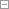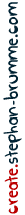# The Mersenne Twister Pseudo Random Number Generator

posted by Stephan Brumme

## Introduction

The Mersenne Twister is often regarded as the fastest pseudo-random number generator which passes almost all statistical tests.

The original C code isn't exactly beautiful, therefore I decided to write my own C++ class.
And for the fun of it, I converted the code to Javascript and added two live demos, too (scroll down).

## Live Demo

This demo will give you the first 10 random numbers generated by the Mersenne Twister.
My C++ implementation returns exactly the same values as the original code written by Makoto Matsumoto and Takuji Nishimura.

These numbers will be computed on the webserver whereas the Javascript code - which generates exactly the same numbers, too - obviously runs in your browser.
Modifying the `seed` value will change the sequence of random numbers. `seed` must be a 32 bit integer. If you leave it empty, 5489 will be used instead.

Seed:
 Seed: C++: Original: Javascript: (in hexadecimal)
I have written a live performance test of the Javascript Mersenne Twister: please scroll down on this page.

## C++ code

The constructor accepts an optional `seed` value. The actual number generation is implemented as a functor.
Your program will look like this:
``` #include "mersenne.h" // create new Mersenne Twister MersenneTwister prng(123456); // generate two random 32-bit numbers int x = prng(); int y = prng(); ```
My implementation generates the same output as the original code when supplied with the same `seed` value.
I got rid of all the `static`s found in the original code which gives you the opportunity to have several independent Mersenne Twisters in your program at the same time.
Moreover, it reduced the risks of nasty concurrency effects in multi-threaded programs.hide mersenne.h - header file ``` // ////////////////////////////////////////////////////////// // mersenne.h // Copyright (c) 2014 Stephan Brumme. All rights reserved. // see http://create.stephan-brumme.com/disclaimer.html // #pragma once #include <stdint.h> /// Mersenne twister pseudo-random number generator /** algorithm invented by Makoto Matsumoto and Takuji Nishimura **/ class MersenneTwister { /// state size enum { SizeState = 624 }; /// internal state uint32_t state[SizeState]; /// offset of next state's word int next; public: /// generate initial internal state MersenneTwister(uint32_t seed = 5489); /// return a random 32 bit number uint32_t operator()(); private: /// create new state (based on old one) void twist(); }; ```hide mersenne.cpp - implementation ``` // ////////////////////////////////////////////////////////// // mersenne.cpp // Copyright (c) 2014 Stephan Brumme. All rights reserved. // see http://create.stephan-brumme.com/disclaimer.html // #include "mersenne.h" /// generate initial internal state MersenneTwister::MersenneTwister(uint32_t seed) : next(0) { state = seed; for (int i = 1; i < SizeState; i++) state[i] = 1812433253UL * (state[i-1] ^ (state[i-1] >> 30)) + i; // let's twist'n'shout ... twist(); } /// return a random 32 bit number uint32_t MersenneTwister::operator()() { // compute new state ? if (next >= SizeState) twist(); // shuffle bits around uint32_t x = state[next++]; x ^= x >> 11; x ^= (x << 7) & 0x9d2c5680; x ^= (x << 15) & 0xefc60000; x ^= x >> 18; return x; } /// create new state (based on old one) void MersenneTwister::twist() { const int M = 397; const int FirstHalf = SizeState - M; // first 624-397=227 words int i; for (i = 0; i < FirstHalf; i++) { uint32_t bits = (state[i] & 0x80000000) | (state[i + 1] & 0x7fffffff); state[i] = state[i + M] ^ (bits >> 1) ^ ((bits & 1) * 0x9908b0df); } // remaining words (except the very last one) for ( ; i < SizeState - 1; i++) { uint32_t bits = (state[i] & 0x80000000) | (state[i + 1] & 0x7fffffff); state[i] = state[i - FirstHalf] ^ (bits >> 1) ^ ((bits & 1) * 0x9908b0df); } // last word is computed pretty much the same way, but i + 1 must wrap around to 0 uint32_t bits = (state[i] & 0x80000000) | (state & 0x7fffffff); state[i] = state[M - 1] ^ (bits >> 1) ^ ((bits & 1) * 0x9908b0df); // word used for next random number next = 0; } ```
Git users: scroll down to the repository link Latest release: August 18, 2014, size: 743 bytes, 32 lines

CRC32: `a028bca2`
MD5: `a0d1de2c02eaa91722b7591c4ca8eb9b`
SHA1: `9e00279dc85bad24eb63379f87cfe20211e9e3ea`
SHA256:`79f195d0f0a1d154995a9fc0c94f8e3ebf17f37169127e28822facbaa17c82db`

Latest release: August 18, 2014, size: 1682 bytes, 66 lines

CRC32: `2f6d248c`
MD5: `0e6b3353d5bae5e7b80f6534d880ec26`
SHA1: `89c1fd6081d6cdc53854a76a8adb7e778374ffea`
SHA256:`8150b7eb8cfe24ed87926fbfa5a0ddc8ee1540cb4a44596a93e8b77206ba3507`

Stay up-to-date:git clone https://create.stephan-brumme.com/mersenne-twister/.git

GitHub mirror:https://github.com/stbrumme/mersenne-twister

If you encounter any bugs/problems or have ideas for improving future versions, please write me an email: create@stephan-brumme.com
There are a few C/C++ implementations available on the internet which might be slightly faster on certain SIMD architectures.
I am not aware of any library written in plain C/C++ that is significantly faster than the code shown above.

## Javascript

The WebKit browser engine used the Mersenne Twister for `Math.random()` (but switched to the faster `XorShift` algorithm recently). However, inside Javascript code there is no way to control the generation of random numbers, for example by defining a seed value - which can be incredibly helpful during testing/debugging because then you can reproduce the same random numbers.

There are only a handful Javascript versions of the Mersenne Twister algorithms and most of them are pretty slow.
My Javascript port computes exactly the same numbers as the C++ version (when using the same `seed` value).
Firefox 31 (Windows) can spit out about 44 million random numbers per second on a three-year-old Intel Core i7 @ 3.4 GHz.
Internet Explorer 11 achieves about 23 million numbers per second on the same computer.
Obviously my Samsung S2 phone (three years old as well) is even slower and can generate only 3.8 million numbers per second (Firefox 31, too).

Live test:
Generate random numbers   alternatively:

Please note that my Mersenne Twister generates 32 bit signed integers whereas the output of `Math.random()` are floating-point numbers between 0 and 1.

And a simple bit distribution heatmap (move your mouse, double-click the heatmap to reset):

 bits 0 - 7 bits 8 - 15 bits 16 - 23 bits 24 - 31
Total of

Usage is pretty straightforward:
``` // load class <script src="http://create.stephan-brumme.com/mersenne-twister/mersenne.js"> </script> <script> // create new Mersenne Twister var prng = new MersenneTwister((new Date).getTime()); // generate two random 32-bit numbers var x = prng.random(); var y = prng.random(); </script> ```
I converted the code from C++ to Javascript by hand instead of using tools like Emscripten.
The most tricky part was coping with bit shifts and the limitation of Javascript's integer system.
Therefore you will find strangely looking symbols like the triple-right-shift `>>>` and explicit integer conversion using the And-Zero idiom (e.g. `state[i] |= 0;`). Git users: scroll down to the repository link Latest release: August 19, 2014, size: 2102 bytes, 75 lines

CRC32: `9c22dfda`
MD5: `641108e6441ec79179742d916f38ad7f`
SHA1: `4f1ad50a16675b5092899774ce0dbc25df9ae98e`
SHA256:`44bc7c23ad2115de39a3fb62e60b25c40c92beb7497c9b0cc91872616ea9a372`

Stay up-to-date:git clone https://create.stephan-brumme.com/mersenne-twister/.git

GitHub mirror:https://github.com/stbrumme/mersenne-twister

If you encounter any bugs/problems or have ideas for improving future versions, please write me an email: create@stephan-brumme.comhide mersenne.js - Javascript version ``` // ////////////////////////////////////////////////////////// // mersenne.js // Copyright (c) 2014 Stephan Brumme. All rights reserved. // see http://create.stephan-brumme.com/disclaimer.html // var MersenneTwister = function(seed) { "use strict"; var state = new Array(624); var next; // if no seed is given, use default value 5489 if (seed == undefined) seed = 5489; // private function: create new state (based on old one) var twist = function() { // first 624-397=227 words for (var i = 0; i < 227; i++) { var bits = (state[i] & 0x80000000) | (state[i + 1] & 0x7fffffff); state[i] = state[i + 397] ^ (bits >>> 1) ^ ((bits & 1) * 0x9908b0df); } // remaining words (except the very last one) for (var i = 227 ; i < 623; i++) { var bits = (state[i] & 0x80000000) | (state[i + 1] & 0x7fffffff); state[i] = state[i - 227] ^ (bits >>> 1) ^ ((bits & 1) * 0x9908b0df); } // last word is computed pretty much the same way, but i + 1 must wrap around to 0 var bits = (state & 0x80000000) | (state & 0x7fffffff); state = state ^ (bits >>> 1) ^ ((bits & 1) * 0x9908b0df); // word used for next random number next = 0; } // fill initial state state = seed; for (var i = 1; i < 624; i++) { var s = state[i - 1] ^ (state[i - 1] >>> 30); // avoid multiplication overflow: split 32 bits into 2x 16 bits and process them individually state[i] = (((((s & 0xffff0000) >>> 16) * 1812433253) << 16) + (s & 0x0000ffff) * 1812433253) + i; // convert to 32 bit unsigned int state[i] |= 0; } // twist'n'shout twist(); // public function: return a random 32 bit number this.random = function() { // compute new state ? if (next >= 624) twist(); // shuffle bits around var x = state[next++]; x ^= x >>> 11; x ^= (x << 7) & 0x9d2c5680; x ^= (x << 15) & 0xefc60000; x ^= x >>> 18; return x; } }; ```
The live demo shows the random numbers as hexadecimal values:hide integer-to-hexadecimal conversion ``` // load class <script src="http://create.stephan-brumme.com/mersenne-twister/mersenne.js"> </script> <script> // create new Mersenne Twister var prng = new MersenneTwister((new Date).getTime()); // generate two random 32-bit numbers var x = prng.random(); var y = prng.random(); </script> ```
Live demo's inner loop must take care of proper Javascript type conversion otherwise full optimization doesn't kick in.
A simple `for`-loop can be two times slower:
``` // prevents Javascript engine from eliminating the whole loop var dummy = 0; // make sure it's an integer var i = numValues | 0; // and go ! while (i-- > 0) dummy ^= prng.random(); // much slower: for (var i = 0; i < numValues; i++) ```